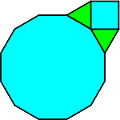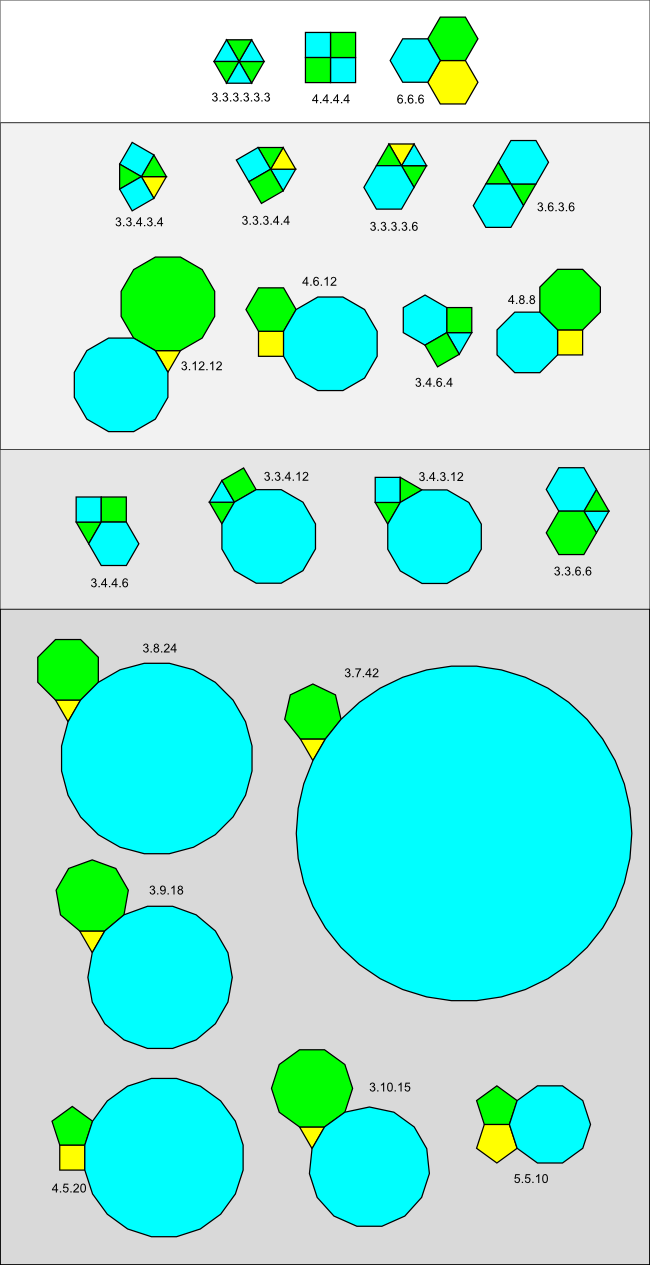# Vertex figuresThis vertex figure has type 3.4.3.12.

The key to classifying regular polygon tilings, as Kepler fully understood, is to look at the way polygons arrange themselves around vertices. The set of polygons that intersect at a single vertex is called a vertex star. If there are polygons adjacent to each edge attached to the vertex in the vertex star, it is called a complete vertex star or a vertex figure. (By definition all the vertex stars in a tiling of the plane are complete.)

Vertex figures are traditionally classified by a vertex type written by placing a dot between the order of each polygon surrounding the vertex.

Kepler used the term congruence for a vertex figure of regular polygons in Harmonices Mundi. Further, he defined a perfect congruence as a congruence that can be extended to a full tiling of the plane by adding more polygons which also intersect in the same vertex type. He defined an imperfect congruence as a congruence that cannot be extended to a full tiling of the plane in this way.

### Completing a vertex star

The angles of all the polygons in a complete vertex star must add up to 2π (360°). The number of ways to complete an incomplete vertex star using regular polygons turns out to be quite limited, as can be seen from the following theorem:

### Gap theorem

Given a vertex star with exactly two edges that do not have two adjacent polygons, if the sum of the angles of the polygons in the vertex star is s, then define the gap angle (the angle of the polygons needed to complete the vertex star) as

2π - s

and the gap ratio g as:

g = (2π - s)/π

If g = 1 (and so the gap angle is π) then the vertex star can be completed using regular polygons in only three ways: by 3 triangles, 2 squares or a triangle and a hexagon.

If g < 1 (and so the gap angle is < π), then the vertex star can be completed using regular polygons if and only if m = 2/(1-g) is an integer.

In this case, the vertex star can be completed by a single m-sided polygon. There is one additional way to complete the vertex star in only three cases:

If g = 2/3, then the vertex star can be completed by two triangles (in addition to a hexagon).
If g = 5/6, then the vertex star can be completed by a triangle and a square (in addition to a dodecagon).
If g = 14/15, then the vertex star can be completed by a triangle and a pentagon (in addition to a 30-gon).

(Note: as proved elsewhere on this site, no complete regular polygon vertex star can contain a triangle and a pentagon or a 30-gon so the third case does not actually occur if the vertex star consists entirely of regular polygons.)

A proof of this theorem is given in this appendix.

### Small polygons

The Gap theorem will be used later in this site and can even be used to classify all possible vertex types but this requires a somewhat tedious consideration of cases, much as Kepler carried out himself in Harmonices Mundi. In the modern computer era we have an easier option.

We can easily write a computer program to search for all the possible combinations of small n-sided polygons (3 <= n <= 12) by examining all the possible sums of the angles in the small polygon table given in the previous section.

Some simple Python code to do this recursive tree search is here.

It turns out that there are 12 ways that small polygon angles can sum to 2π. For four of these cases, there are two distinct ways to order the polygons, giving 16 different vertex types in all. These are all given in the chart displayed at the bottom of this page.

### Large polygons

What about vertex stars containing large polygons?

Here a crucial observation allows us to finish our classification: a vertex figure of regular polygons can include only one large polygon.

Why? Notice that 3.12.12 is a vertex type. If we increase the order of a polygon beyond 12, we also increase its edge angle. But this must *reduce* the edge angles of the other polygons in the vertex figure since the sum must still be 2π. Since a large polygon is of order larger than 12 and 3.12.12 is a vertex type, this means that if a large polygon occurs twice in a vertex figure, the sum of the remaining polygons must be smaller than a triangle. But the triangle has the smallest possible regular polygon angle so this is impossible,

Given this information, determining the list of vertex types involving large polygons is also a matter of simple arithmetic.

Suppose sπ is the sum of the angles of all the small polygons and the large polygon has order m.

Then sπ + (m-2)π/m = 2π and so:
sπ = 2π - (m-2)π/m

We know that the angle (m-2)π/m is greater than the angle for the polygon of order 12 (10π/12) and less than π since no polygon can have edge angles greater than π (180°).

From the last equation, this means that sπ must lie between π and 2π - 10π/12 = 22π/12.

Again this is a simple search of all the possible small angle sums.

Some simple Python code to do this recursive tree search is here.

Here there are six solutions, all with three polygons. For each solution we can solve for m given the sum of small angles sπ.

(m-2)π/m = 2π - sπ

so, solving for m:

m = 2/(s-1)

Here are the solutions where s1 and s2 are the orders of the two small polygons and m is the calculated order of the large polygon.

The six large solutions
s1 s2 m
3 7 42
3 8 24
3 9 18
3 10 15
3 11 13.2
4 5 20

Obviously there is no regular polygon with 13.2 sides, so this gives five additional vertex types.

### Kepler's mistake

Kepler's largest error in his vertex classification concerned the larger polygons. In Section XX of Book II of Harmonices Mundi, he wrote:

Nor can a trigon [triangle] angle join up with one angle of a heptagon or of an octagon or of an enneagon [nonagon], for the gap left for the third kind of angle would be forty twenty-firsts of a right angle, or eleven sixteenths or sixteen ninths, none of which is the angle of a regular figure.

Kepler was correct in his arithmetic for two of the items at least, but incorrect in his geometry, for a right angle is (π/2) and:

• the angle between the edges of a 42-gon is (40/42)π = (40/21)(π/2), which completes the 3.7.42 vertex type
• the angle between the edges of a 24-gon is (22/24)π = (22/12)(π/2) = (11/6)(π/2) which completes the 3.8.24 vertex type
• the angle between the edges of an 18-gon is (16/18)π = (16/9)(π/2) which completes the 3.9.18 vertex type

I suspect that the word "sixteenths" in the above translation is a typo and Kepler meant "sixths" which would have made his arithmetic (if not his geometry) completely correct. I don't know enough Latin to check the original version to see if the error is in the original or in the translation.

Kepler was interested in the "perfect congruences" so the error made no real difference to his conclusion as he got all of the "perfect congruences" correct.

### Classification chart

Combining the 16 small and 5 large vertex types gives us 21 vertex types in all. Any vertex in an edge-to-edge tiling of regular polygons must have one of these types.

I have divided the chart below into four different vertex families. Each of the first three families gives rise to a distinct kind of tiling as explained in the following pages of this site. The fourth vertex family, which includes 5.5.10 and all the large polygons, can never occur in any tiling of regular polygons. A proof for this is given later in this site.### A note about vertex types

Mathematicians consider two tilings to be identical if one can be converted into the other by scaling, translation, rotation or reflection. The same is true for vertex figures. This means that for two vertex types, if one can be converted into the other by cyclic permutation or reversal, then they are considered to be identical.

For example,

3.3.4.12 = 3.4.12.3 = 4.12.3.3 = 12.3.3.4 by cyclic permutation, and

3.3.4.12 = 12.4.3.3 by reversal.

However, 3.3.4.12 is not the same as 3.4.3.12 because the two types cannot be converted into each other by cyclic permutation or reversal.

Vertex types are usually written by selecting from the list of identical types the one that has the most number of lowest order polygons first. For example, the vertex type in the above example would be written 3.3.4.12 no matter how the vertex figure is displayed - even if it is a mirror image. This means that the starting point or the order one chooses to go around the vertex figure (clockwise or counter-clockwise) is irrelevant as it does not affect the vertex type.